# Quiz 6: Service Quality

Control Charts are graphs that show if a sample is within statistical control limits. Here, control limits are the upper and lower bands of a control chart. X-bar charts are used to check the productivity of the process with respect to its average performances and predetermined targets. R-charts are used to check and monitor the variability in the process. To prepare X-bar chart and R-bar chart for complaints, begin by calculating the average complaints and range for each crew, as shown below: The range is calculated by subtracting the minimum value from the maximum value for each crew. For instance, for crew A, Range = 2 - 0 = 2.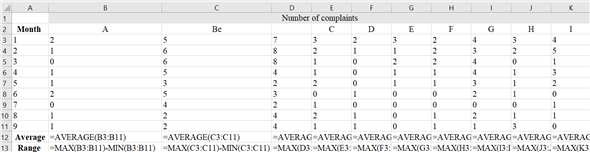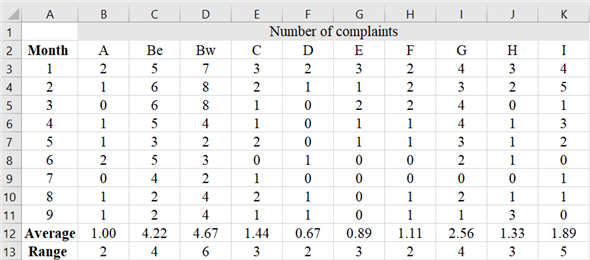Note : The formula in cell B12 and B13 have been copied till cell K12 and K13 respectively. Now, compute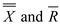.Now, construct the control limits for thecharts. The following factors for n = 9 are taken from the Variable Control and Chart Constraints :The upper and lower control limits for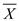chart are calculated using the following formulae:Now, enter the following details in Excel to construct thechart.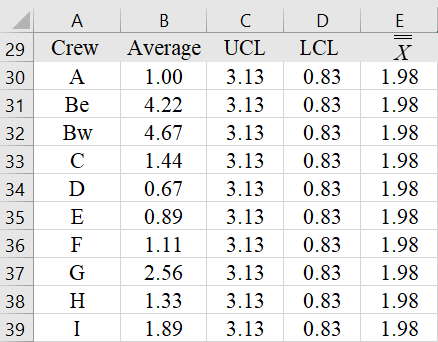Select the cell range A30:E39. Go to the Insert Tab and choose the line chart from the options. Thechart for complaints is as follows: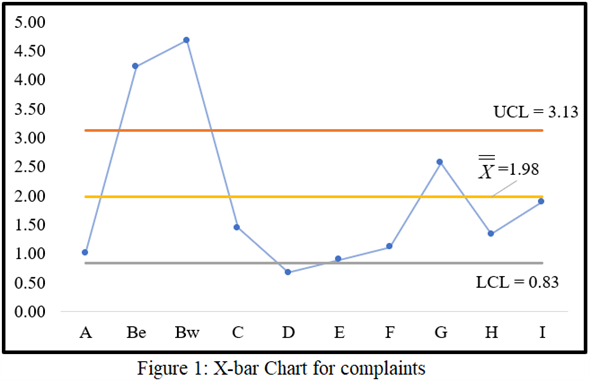The upper and lower control limits for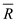chart are calculated using the following formulae:Now, enter the following details in Excel to construct thechart.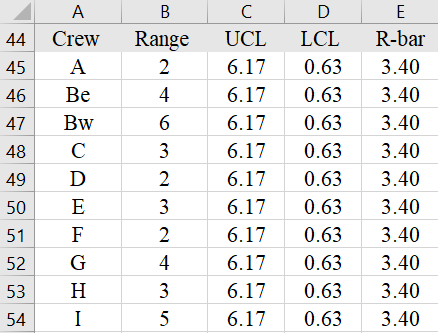Select the cell range A45:E54. Go to the Insert Tab and choose the line chart from the options. Thechart for complaints is as follows: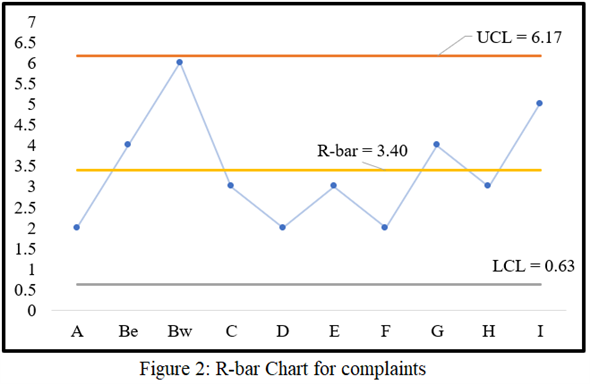Now, repeat the above steps to construct the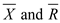for customer ratings. The average ratings and range for each crew are calculated below: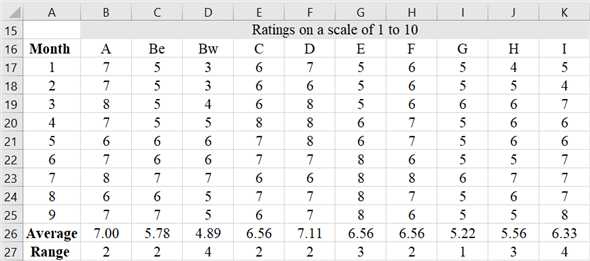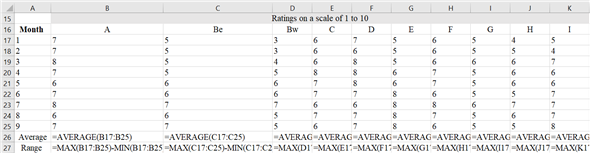Note : The formula in cell B26 and B27 have been copied till cell K26 and K27 respectively. Now, compute.Now, construct the control limits for thecharts. The following factors for n = 9 are taken from the Variable Control and Chart Constraints :The upper and lower control limits for thecharts are calculated using the following formulae:Now, enter the following details in Excel to construct thechart.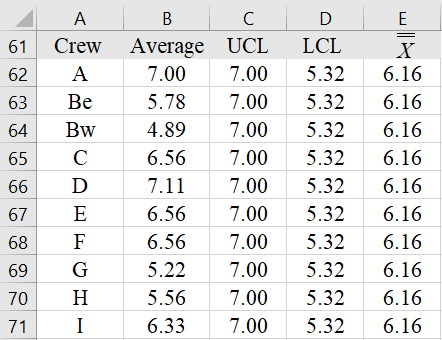Select the cell range A62:E71. Go to the Insert Tab and choose the line chart from the options. Thechart for ratings is as follows: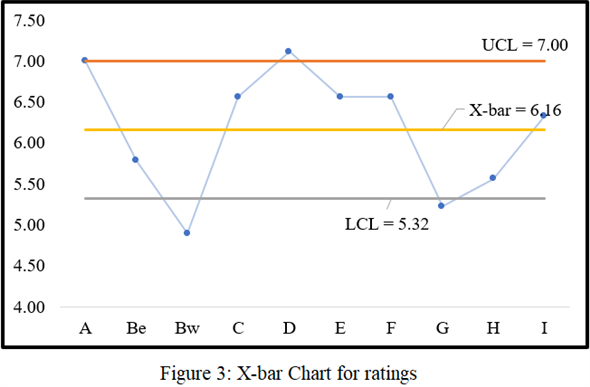The upper and lower control limits for thechart are calculated using the following formulae:Now, enter the following details in Excel to construct thechart.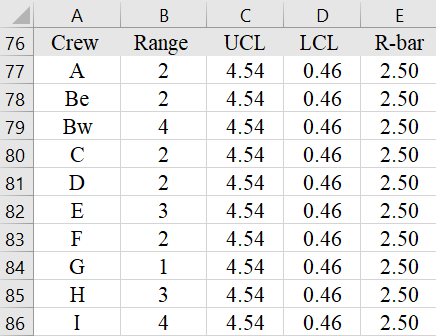Select the cell range A77:E86. Go to the Insert Tab and choose the line chart from the options. Thechart for ratings is as follows: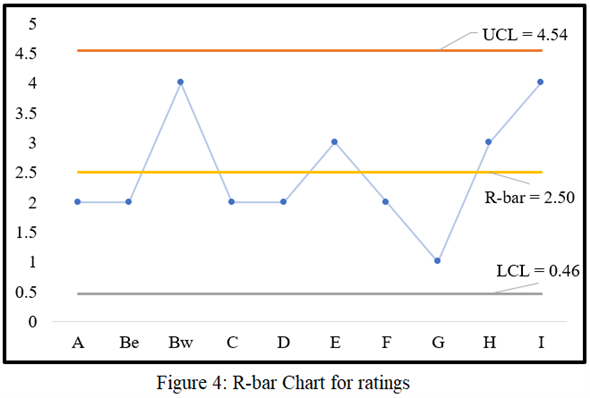From Figure 1 i.e. the X-bar chart for complaints , it can be observed that crews Be and Bw receive more than usual complaints as compared to others. The upper limit for complaints in the X-bar charts is 3.13 complaints over the 9 months, however these two have received more than 4 complaints over this period. In addition, the service quality of crew D is exceptional among all since it received least number of complaints. The service quality of the remaining crews is good as their number of complaints pertains to the control limits of the process. From Figure 2 i.e. the R-bar chart for complaints , it can be observed that the complaints are not variating from the control limits which reflects stability in the customer service quality. Since the range chart is in control, the control limits on the X-bar chart are reliable. From Figure 3 i.e. the X-bar chart for ratings, it can be observed that crews Bw and G have the poorest ratings among all. This reflects non-satisfactory service quality over the 9-month period. In addition, crew D has the highest average rating for this period reflecting best service among all. Similarly, in Figure 4 i.e. the R-bar chart for ratings, it can be observed that the ratings are not variating from the control limits which reflects stability in the customer service quality. Since the range chart is in control, the control limits on the X-bar chart are reliable. From the entire analysis, it is inferred that crew D has the best service quality as compared to others in this 9-month period.